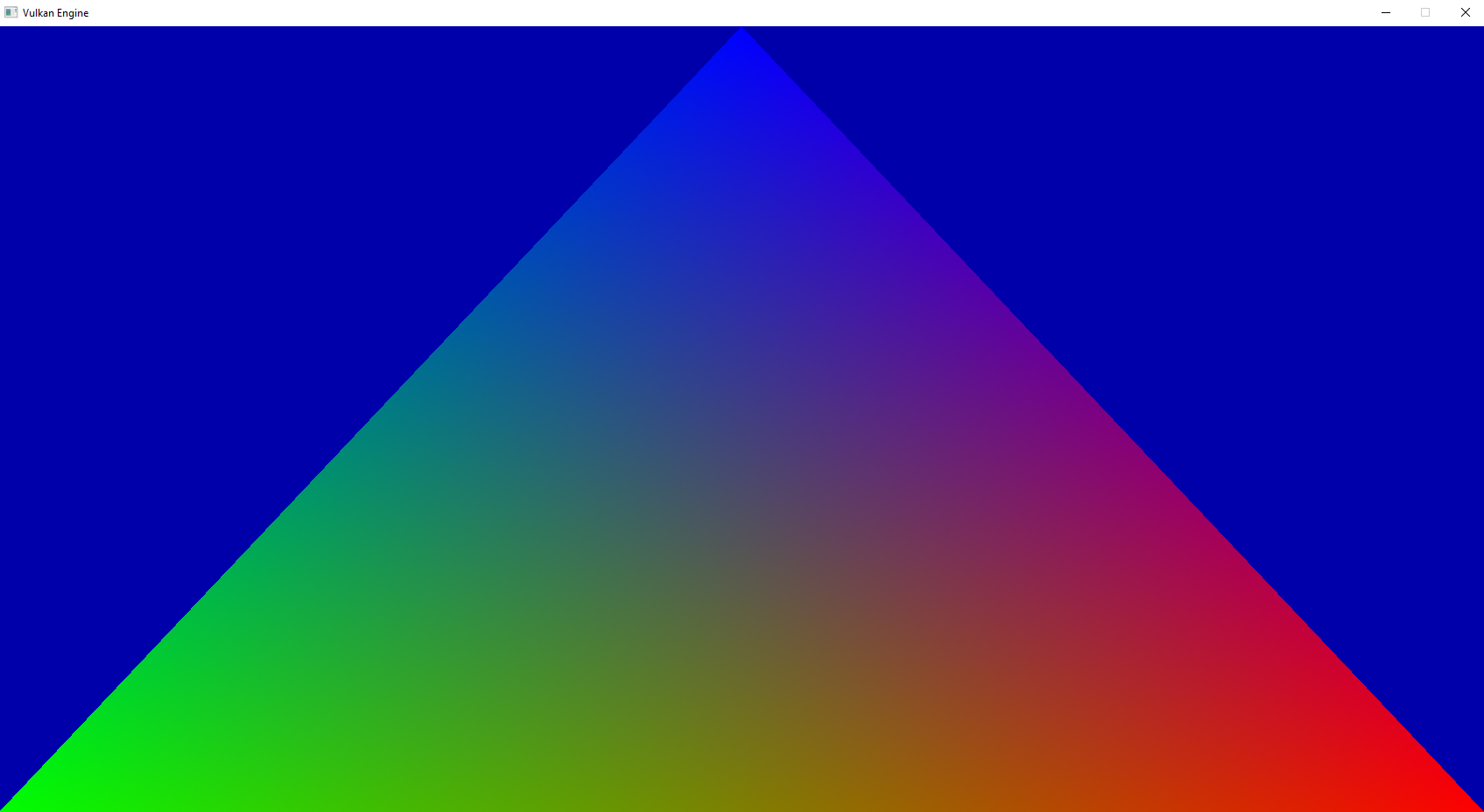We have been able to render a red triangle, but this is too boring. Now, we will add more color to it, converting it into the classic RGB triangle.

To do that, we are going to need to pass more data from the vertex shader to the fragment shader. At the moment we only pass the position, which is a default Vulkan variable, but there isn’t a color variable we can use, so we need to make our own.

All shader stages can pass data between them by using input and output variables. If in the vertex shader we create an output variable, we will be able to read it on the fragment shader as an input variable.

A very important detail, is that when compiling a pipeline which uses this sort of input and output variables, they always have to match. If there is a mismatch, the pipeline compilation will fail.

To create an output variable, we do this on the vertex shader.

``````layout (location = 0) out vec3 outColor;
``````

`layout(location = 0)` is a decorator that lets us add “extra data” to variable declarations and other things in GLSL. In here, we are declaring `location = 0`, which means that this variable of type vec3 will be on the number 0 slot.

To read the variable from pixel stage, we do this.

``````layout (location = 0) in vec3 inColor;
``````

The names of the variables do not need to match. On the vertex shader we are calling it `outColor`, but on the fragment shader we are calling it `inColor`. What does need to match, is the Location decorator and the type of the variable. In this case, it’s a location 0 vec3 variable, so things match.

We can output most default types from the vertex shader into the fragment shader, but be careful with it, as the more data you need to pass between stages, the more overhead you will have. Old GPUs even have a very small number of how many variables can be passed.

Now that we know how to pass data from the vertex shader into the fragment shader, let’s use it to create a colorful triangle.

Create a new shader, called `colored_triangle.vert`. It will be mostly the same as the `triangle.vert` shader, but with colors.

``````//output variable to the fragment shader
layout (location = 0) out vec3 outColor;

void main()
{
//const array of positions for the triangle
const vec3 positions = vec3(
vec3(1.f,1.f, 0.0f),
vec3(-1.f,1.f, 0.0f),
vec3(0.f,-1.f, 0.0f)
);

//const array of colors for the triangle
const vec3 colors = vec3(
vec3(1.0f, 0.0f, 0.0f), //red
vec3(0.0f, 1.0f, 0.0f), //green
vec3(00.f, 0.0f, 1.0f)  //blue
);

//output the position of each vertex
gl_Position = vec4(positions[gl_VertexIndex], 1.0f);
outColor = colors[gl_VertexIndex];
}
``````

In the same way as we do with positions, we are declaring a const array of colors, Red, Green, and Blue, and then outputting them to the outColor variable.

Create a new shader, called `colored_triangle.frag`.

``````#version 450

layout (location = 0) in vec3 inColor;

//output write
layout (location = 0) out vec4 outFragColor;

void main()
{
//return color
outFragColor = vec4(inColor,1.0f);
}
``````

We can now read the color in the fragment shader, and output it as the final color.

Make sure to rebuild the Shaders target in Cmake, as we need it to compile the new things. It shouldn’t have any error

## Changing the shaders in code

Now, let’s change the shaders used in the code, to switch the red triangle shader into a colored triangle shader.

On the code that loads the triangle shaders, we change the filenames

`````` VkShaderModule triangleFragShader;
{
std::cout << "Error when building the triangle fragment shader module" << std::endl;
}
else {
}

{
std::cout << "Error when building the triangle vertex shader module" << std::endl;

}
else {